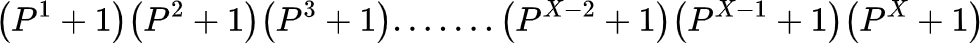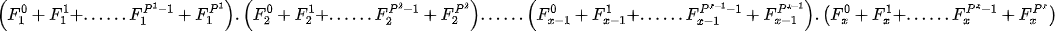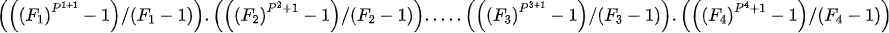# How to find the number of divisors and sum of divisors of a number

## Introduction

Finding the number of divisors and the sum of divisors of a number is a very important concept for programming. Even though it is easy to find the number of divisors and the sum of divisors of a number if it is small, finding the number and sum of divisors of a large number will be difficult.

So let's first begin with finding the number of divisors of a number, then we will learn about finding the sum of divisors of a number.

## The number of divisors of a number

To find the number of divisors of a number, we start by finding the prime factorization of the number, as all the divisors of a number will be a subset of the number's prime factorization. In simple words, if we can devise a way to find all the subset of prime factorizations of a number, we can find the number of divisors of that number.

Let's say we have a number with x terms in its prime factorization. Then to find the total number of divisors of this number, we will have to find the total number of ways to select any number of terms from these x terms or the total number of subsets of x. It would have been easier because the total number of subsets of a set is 2no. Of elements in the set, but here the x might have some repeated terms. So we first find the prime factorization of a number, then represent the number in terms of distinct prime factors. For example- we can represent 36 as 22.32

Now, suppose we have a number F which appears p times in the prime factorization of a number X, then it can appear in subsets in p+1 ways, i.e.,So, for a number,We can say that each distinct factor Fhas Pi+1 ways to appear in subsets. So the total number of divisors of a number Y=## The sum of divisors of a number

To find the sum of all the divisors of a number, we will start with the formulae we used to find the number of divisors.

As we know, if a number F appears p times in the prime factorization of a number X, it can appear in subsets in p+1 ways, i.e.,Now, if we multiply the set of choices of each factor, we will get a set of all possible divisors.

For example, if we have a number with two factors, then all the terms in the productwill form the set containing all the divisors.

Since we need the sum of all the divisors of a number, we will find the product of the sum of all the choices for each factor. For the above example- thewill represent the sum of all the divisors of a number.

So, for any number,the sum of all the divisors of a number will beIn this expression, we can observe that each term in the product is a GP. and for any GP, the sum of all the terms isUsing this for every term in the formulaWe will get the result as-1. How can you find the total no. of divisors of a number?
To find the total number of divisors of a number, we first find the prime factorization of the number and then find each distinct prime number's exponents. Then we add one to all the exponents and then find their product. The product is the number of divisors of a number.

2. What is the multiplicative identity?
Multiplicative identity states that the product of any number with 1 is the number itself. Thus, we can also say that one is a divisor of every number.

3. How do you find the number of odd divisors of a number?
To find the number of odd divisors of a number, we first find the prime factorization of the number and then find each distinct prime number's exponents. Now, we remove even prime numbers in it. Then we add one to all the exponents of odd prime numbers and then find their product. The product is the number of odd divisors of a number.

4. How do you find the number of odd divisors of a number?
Since we know how to find the total number of divisors of a number and odd divisors of a number, the difference of both will be the even divisors of a number.

5. What are the proper divisors of a number?
The proper divisors of a number refer to the divisors, which are smaller than the number itself.

## Key takeaways

In this blog, we have learned the formula for the number of divisors of a number and the sum of divisors of a number-

• We learned about the formula to find the number of divisors of a number because any divisor of a number will be formed by selecting some factors from the prime factorization of the number. Then we found that the number of choices for each factor in forming another divisor is one more than the number of times it appears in the prime factorization of the number. Thus, the total number of choices for each factor gives us the total number of divisors of a number.
• Then we learned about the formula to find the sum of divisors of a number using the similar concept we used for finding the number of divisors of a number. Since the product of all the choices for each factor for forming a divisor gives the total number of divisors. So, we find the product of the sum of the total number of choices for each distinct factor in the prime factorization of the number, which gives us the sum of all the number's divisors.

Visit here to learn more about algorithms in programming. You can also practice similar questions on Code studio.